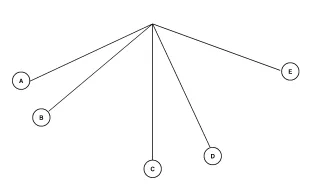# Problem: The kinetic energy of the pendulum bob in figure 15-1 increases the most between locationsa. A and B.b. B and D.c. A and C.d. C and D.

###### FREE Expert Solution

We are required to compare the changes in kinetic energy as the pendulum moves across positions.

Law of conservation of energy:

$\overline{){{\mathbf{K}}}_{{\mathbf{i}}}{\mathbf{+}}{{\mathbf{U}}}_{{\mathbf{i}}}{\mathbf{+}}{{\mathbf{W}}}_{{\mathbf{nc}}}{\mathbf{=}}{{\mathbf{K}}}_{{\mathbf{f}}}{\mathbf{+}}{{\mathbf{U}}}_{{\mathbf{f}}}}$, where Wnc is the work done by non-conservative forces such as friction.

82% (409 ratings)###### Problem Details

The kinetic energy of the pendulum bob in figure 15-1 increases the most between locations

a. A and B.

b. B and D.

c. A and C.

d. C and D.